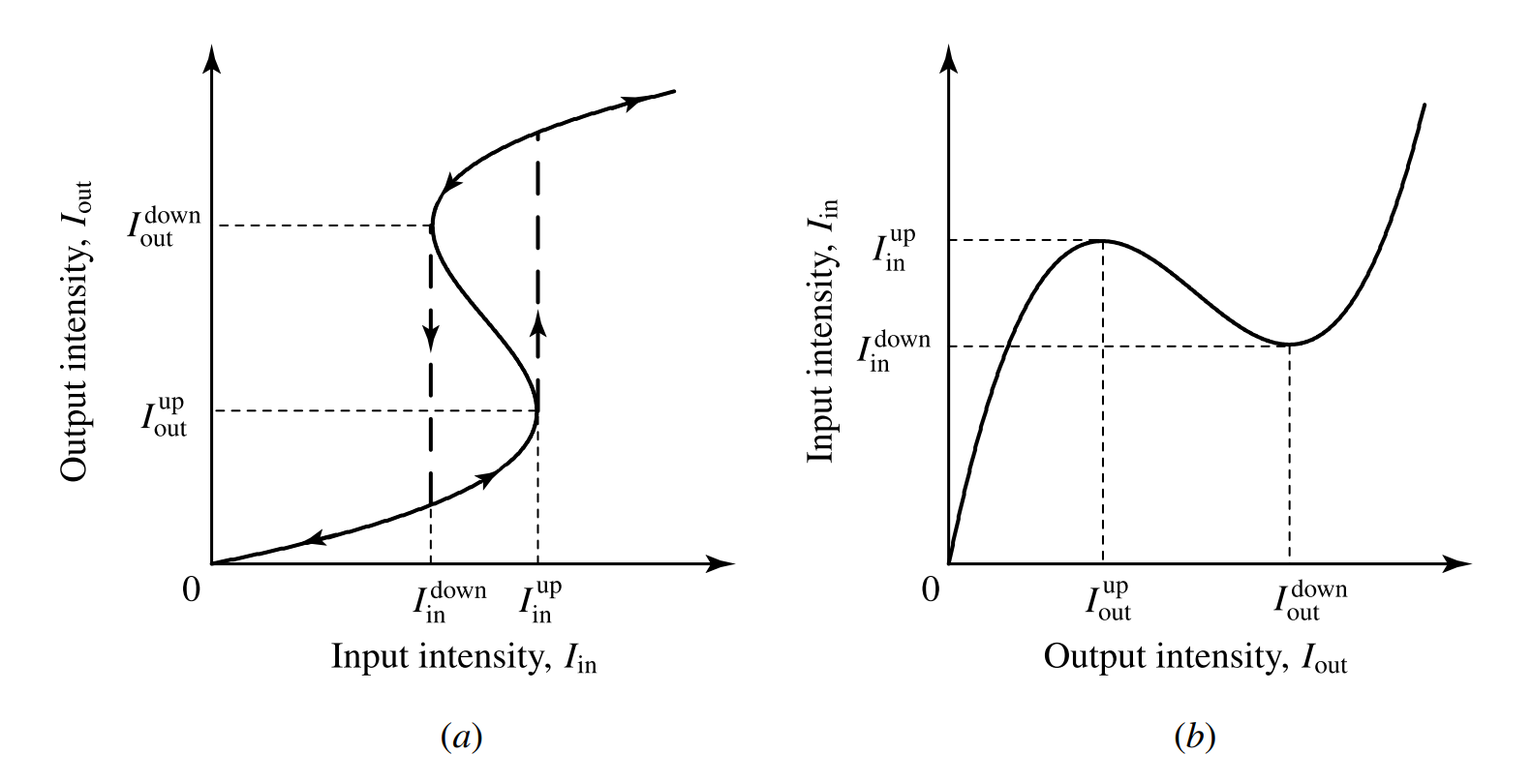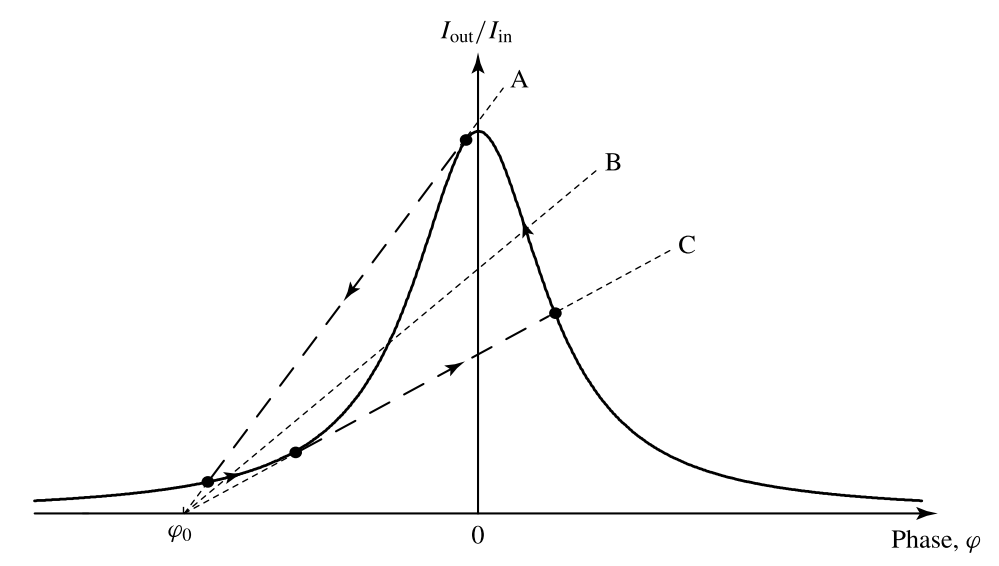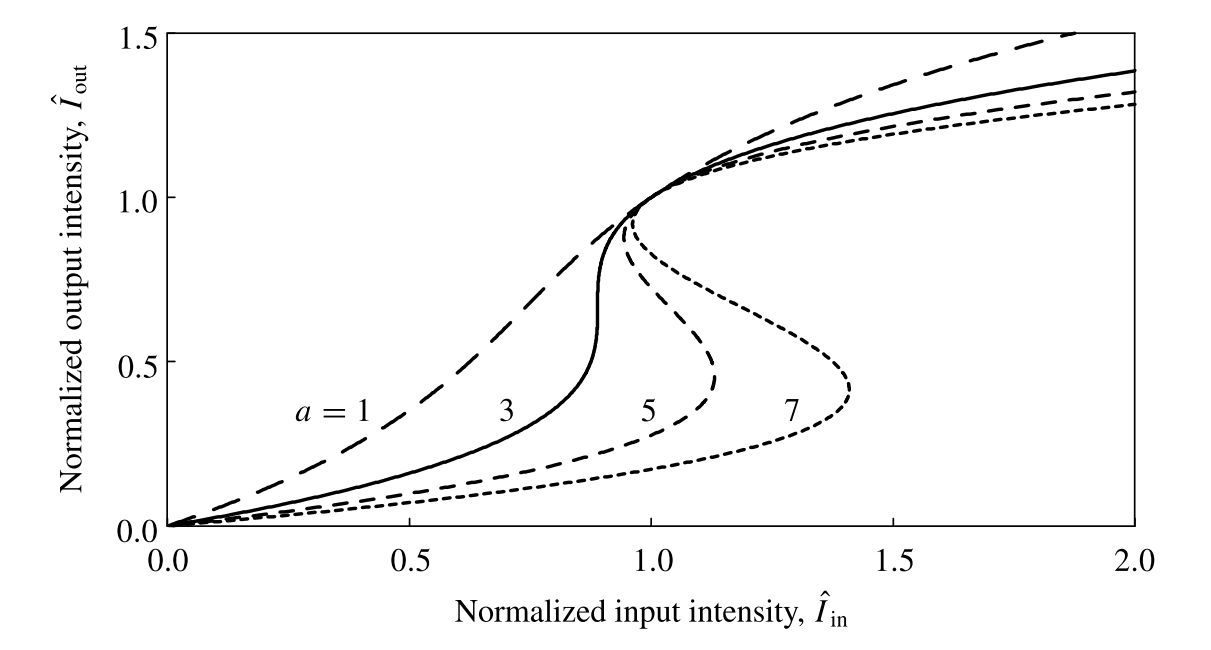# Bistable optical devices

This is a continuation from the previous tutorial - nonlinear optical modulators and switches.

A bistable device has two stable output states under one input condition. Because of this binary feature, bistable devices can be used for many digital operations, such as switches, memories, registers, and flip-flops. Bistable electronic circuits and devices have become indispensable components in a wide range of application that require the storage of binary information. Bistable optical devices can be important for their applications as optical logic, memories, and analog-to-digital converters in optical signal processing systems. In addition, they can also be used as optical pulse discriminators and optical power limiters.

The output parameter of a bistable device is a multivalued function of its input parameter. Any system with such a multivalued characteristic is by definition a nonlinear system. Therefore, optical nonlinearity is absolutely required for a bistable optical device.

Optical nonlinearity alone is not sufficient for bistability, however. As seen from earlier tutorials, the propagation characteristics of an optical beam through a nonlinear medium vary nonlinearly but also monotonically with the beam intensity. Bistability is not possible with monotonic nonlinearity alone because a monotonic characteristic does not lead to a multivalued dependence of the output on an input parameter. The required non-monotonic characteristics for optical bistability can be made possible only with proper feedback.

The necessary conditions for optical bistability are optical nonlinearity and positive feedback. Depending on whether the optical nonlinearity responsible for the bistable function comes from the real or the imaginary part of a nonlinear susceptibility, a bistable optical device can be classified as either dispersive or absorptive. In some devices, this distinction is not clear, however, because both refractive and absorptive nonlinear mechanisms may be present. Depending on the type of feedback, a bistable optical device can also be classified as either intrinsic or hybrid. In an intrinsic bistable device, both the interaction and the feedback are all optical. In a hybrid bistable device, electrical feedback is used to modify the optical interaction, thereby creating an artificial optical nonlinearity.

Figure 9-25(a) below shows a generic characteristic for intensity bistability of a bistable optical device. For each input intensity within the range between $$I_\text{in}^\text{down}$$ and $$I_\text{in}^\text{up}$$, there are three values for the output intensity. Only the two values that lie on the upper and the lower branches of the curve are stable output values. The one that lies on the middle branch is unstable because the middle branch has a negative slope: $$\text{d}I_\text{out}/\text{d}I_\text{in}\lt0$$. When the input intensity is gradually increased from zero, the output intensity traces the lower branch of the curve until the input intensity reaches the up-transition point at $$I_\text{in}^\text{up}$$, where the output makes a sudden jump to the upper branch. Once the system is in a state that lies on the upper branch, it can be brought back to the lower branch only when the input intensity is lowered to the down-transition point at $$I_\text{in}^\text{down}$$. If the input intensity is set at a value within the bistable region, the output can be in either stable state depending on the history of the system. With a proper external excitation, it can be switched from one of the stable states to the other. Otherwise, it stays in one state indefinitely.Figure 9-25. Generic characteristic for intensity bistability (a) plotted with output intensity $$I_\text{out}$$ as a function of input intensity $$I_\text{in}$$ and (b) plotted with $$I_\text{in}$$ as a function of $$I_\text{out}$$.

We see from Figure 9-25(a) that the slope of the characteristic curve for bistability changes sign at both up- and down-transition points. This fact can be exploited to find the condition for bistability and the transition points. Though $$I_\text{out}$$ is a multivalued function of $$I_\text{in}$$, $$I_\text{in}$$ is a single-valued function of $$I_\text{out}$$. Therefore, it is convenient to express $$I_\text{in}$$ as a function of $$I_\text{out}$$, as shown in Figure 9-25(b). From the curve shown in Figure 9-25(b), we find that the condition for the existence of a bistable region is the existence of a region of negative slope, $$\text{d}I_\text{in}/\text{d}I_\text{out}\lt0$$, between regions of positive slope. Because both $$I_\text{in}$$ and $$I_\text{out}$$ are real and positive quantities, this condition can be satisfied only when the relation

$\tag{9-157}\frac{\text{d}I_\text{in}}{\text{d}I_\text{out}}=0$

has two nondegenerate real and positive solutions. These two solutions correspond to the two transition points $$(I_\text{in}^\text{up},I_\text{out}^\text{up})$$ and $$(I_\text{in}^\text{down},I_\text{out}^\text{down})$$, as can be seen by an examination of Figures 9-25(a) and (b).

In principle, it is possible to construct bistable optical devices using a variety of different nonlinear effects discussed in the nonlinear optical interactions tutorial if the device parameters are properly chosen. In practice, however, the nonlinear optical media that are most commonly used for bistable devices are either non-absorptive Kerr media, for the dispersive type, or saturable absorbers, for the absorptive type.

A simple bistable optical device of the intrinsic type can be constructed by placing a nonlinear optical medium inside a Fabry-Perot cavity, as shown in Figure 9-26(a) below, or inside a ring cavity, as shown in Figure 9-26(b) below.Figure 9-26. Intrinsic bistable optical devices using optical feedback in the configurations of (a) a Fabry-Perot cavity and (b) a ring cavity.

The mirrors of the cavity provide the needed optical feedback to the nonlinear optical interaction. The only difference between the two configurations in Figure 9-26 is that the optical wave in a Fabry-Perot cavity travels through the nonlinear medium twice in each round trip and forms a standing wave pattern, but the wave in a ring cavity is a traveling wave that travels through the nonlinear medium only once in each round trip. Otherwise, the basic principle and the characteristics of optical bistability are the same for the two configuration. Other optical feedback configurations for intrinsic bistable optical devices are based on this concept as well.

In the following, we consider bistable devices that have the configuration of the Fabry-Perot cavity shown in Figure 9-26(a). For simplicity, we assume that the mirrors of the Fabry-Perot cavity are identical and lossless. The mirrors have a real reflection coefficient $$r$$, which can be either positive or negative, but their transmission coefficient $$t$$ can be complex because of the finite thickness of the mirrors. The intensity reflectance is $$R=r^2$$, and the intensity transmittance is $$T=|t|^2=1-R$$, for $$R+T=1$$. We also assume that the nonlinear medium fills up the entire space inside the Fabry-Perot cavity of length $$l$$. We ignore transverse spatial variations by considering only plane optical waves. When the steady state is reached, the forward-traveling field $$\mathcal{E}_\text{f}$$, and the backward-traveling field $$\mathcal{E}_\text{b}$$, inside the cavity satisfy the following relations at the input end, $$z=0$$:

$\tag{9-158}\mathcal{E}_\text{f}(0)=t\mathcal{E}_\text{in}+r\mathcal{E}_\text{b}(0)$

$\tag{9-159}\mathcal{E}_\text{b}(0)=r\mathcal{E}_\text{f}(0)\text{e}^{\text{i}2kl-\alpha{l}}$

where $$k$$ and $$\alpha$$ are the propagation constant and the absorption coefficient, respectively, in the medium. At the output end, $$z=l$$, we have

$\tag{9-160}\mathcal{E}_\text{out}=t\mathcal{E}_\text{f}(l)=t\mathcal{E}_\text{f}(0)\text{e}^{\text{i}kl-\alpha{l}/2}$

Using these relations, we find that

$\tag{9-161}\mathcal{E}_\text{out}=\frac{t^2\text{e}^{\text{i}kl-\alpha{l}/2}}{1-r^2\text{e}^{\text{i}2kl-\alpha{l}}}\mathcal{E}_\text{in}$

which gives the following relation between the input and output intensities:

$\tag{9-162}I_\text{out}=\frac{(1-R)^2\text{e}^{-\alpha{l}}}{(1-R\text{e}^{-\alpha{l}})^2+4R\text{e}^{-\alpha{l}}\sin^2kl}I_\text{in}$

Dispersive bistable optical devies

We first consider dispersive bistability in a Fabry-Perot cavity filled with a nonlinear medium that has an intensity-dependent index of refraction due to the optical Kerr effect. For simplicity, we ignore the standing wave pattern in the cavity and take the average intracavity intensity $$I_\text{c}\approx{I}_\text{f}+I_\text{b}\approx2I_\text{out}/(1-R)$$. The intensity-dependent index of refraction is

$\tag{9-163}n=n_0+n_2I_\text{c}\approx{n}_0+\frac{2n_2I_\text{out}}{1-R}$

Then, the total phase shift over a round trip in the cavity can be expressed as

$\tag{9-164}2kl=2\frac{\omega{n}}{c}l=\frac{2n_0\omega{l}}{c}+\frac{4n_2\omega{l}}{c(1-R)}I_\text{out}=2m\pi+\varphi$

where $$m$$ is a properly chosen integer such that

$\tag{9-165}\varphi=\varphi_0+\varphi_2I_\text{out}$

for $$|\varphi_0|\lt\pi$$ and

$\tag{9-166}\varphi_2=\frac{4n_2\omega{l}}{c(1-R)}=\frac{8\pi{n}_2l}{\lambda(1-R)}$

Note that $$\varphi_0$$ is a bias phase that can be chosen at will by slightly varying the cavity length $$l$$ for a given optical frequency $$\omega$$ or by varying the optical frequency for a fixed cavity length.

For the device under consideration, we can rearrange (9-162) as

$\tag{9-167}\frac{I_\text{out}}{I_\text{in}}=\frac{F^2/F_0^2}{1+4(F^2/\pi^2)\sin^2(\varphi/2)}$

where

$\tag{9-168}F=\frac{\pi\sqrt{R\text{e}^{-\alpha{l}}}}{1-R\text{e}^{-\alpha{l}}}$

is the finesse of a generic lossy Fabry-Perot cavity, and

$\tag{9-169}F_0=\frac{\pi\sqrt{R}}{1-R}$

is the finesse of a lossless Fabry-Perot cavity.

The characteristic described by (9-167) has resonance peaks at $$\varphi=0,\pm2\pi,\pm4\pi,\cdots$$, each of which has the same characteristics as those of the peak shown in Figure 9-27 below.Figure 9-27. Graphic illustration of the bistable characteristic of a dispersive bistable device with a Fabry-Perot cavity. The peaked curve is the transmission characteristic of the Fabry-Perot cavity at a resonance condition. Other resonance peaks are far away and are not shown. Line A represents the input intensity at $$I_\text{in}^\text{down}$$. Line B represents an input intensity in the bistable region. Line C represents the input intensity at $$I_\text{in}^\text{up}$$.

For $$\varphi$$ being intensity dependent as given in (9-165), the system can exhibit intensity bistability under proper conditions. A graphic solution can be obtained by expressing (9-165) in the form of

$\tag{9-170}\frac{I_\text{out}}{I_\text{in}}=\frac{\varphi-\varphi_0}{\varphi_2I_\text{in}}$

and plotting it as straight lines for various values of $$I_\text{in}$$ to find the intersections between these lines and the curve representing (9-167).

An example of the graphic solution for $$\varphi_0\lt0$$ and $$n_2\gt0$$ is shown in Figure 9-27 above. It can be seen from this illustration that up-transition corresponds to line C, which is tangent to the curve at its heel, whereas down-transition is described by line A, which is tangent to the curve near its peak.

Analytical solution is possible if $$|\varphi|\lt1$$ so that $$\sin^2(\varphi/2)\approx\varphi^2/4$$. Then, by combining (9-165) and (9-167), we have

$\tag{9-171}\frac{F^2}{F_0^2}I_\text{in}=\left[1+\frac{F^2}{\pi^2}(\varphi_0+\varphi_2I_\text{out})^2\right]I_\text{out}$

By demanding that $$\text{d}I_\text{in}/\text{d}I_\text{out}=0$$ has two nondegenerate, real and positive solutions for $$I_\text{out}$$, we find that the conditions for bistability under the assumption that $$|\varphi|\lt1$$ are

$\tag{9-172}\varphi_0n_2\lt0$

and

$\tag{9-173}|\varphi_0|\gt\frac{\sqrt{3}\pi}{F}$

Once the conditions for bistability are satisfied, the up- and down-transition points, as well as the region of bistability, are found using the two nondegenerate solutions for $$I_\text{out}$$.

Figure 9-28 below shows the characteristics of this dispersive device for a few different values of the characteristic parameter $$a=F^2\varphi_0^2/\pi^2$$ under the condition that (9-172) is satisfied. It can be seen that bistability exists only when $$a\gt3$$ so that (9-173) is satisfied.Figure 9-28. Characteristics of a dispersive nonlinear device with an optical Kerr medium in a Fabry-Perot cavity, where $$\hat{I}_\text{in}=-F^2\varphi_2I_\text{in}/(F_0^2\varphi_0)$$ and $$\hat{I}_\text{out}=-\varphi_2I_\text{out}/\varphi_0$$. Bistability exists only when $$\varphi_2/\varphi_0\lt0$$ and $$a=F^2\varphi_0^2/\pi^2\gt3$$. The solid curve represents the threshold condition for bistability at $$a=3$$.

There is a threshold input intensity, $$I_\text{th}$$, required for bistability to be possible in a device. This threshold input intensity is

$\tag{9-174}I_\text{th}=\frac{\sqrt{3}\lambda(1-R)}{9F|n_2|l}\frac{F_0^2}{F^2}$

This bistability threshold intensity corresponds to the switching intensity for $$a=3$$. If the input intensity is limited to below this threshold, the device can never reach its switching point even when both $$F$$ and $$\varphi_0$$ are made large enough so that $$a\gt3$$. When the input intensity exceeds this threshold, the device can enter its bistable regime for a properly chosen value of $$a\gt3$$. However, the minimum input intensity required for a given device to operate properly in its bistable states increases as the value of $$\varphi_0$$ and, correspondingly, the value of $$a$$ for a given finesse $$F$$ increase.

Ideally for a dispersive bistable device, the medium should be completely lossless. In practice, however, there are always some absorption or scattering losses in the medium. Such losses in a dispersive device are usually very small so that $$\alpha{l}\ll1$$. Though the losses are small, they can reduce the finesses of the cavity, thus significantly increasing the input intensity required for the operation of the device.

Example 9-16

A vertical cavity InGaAsP bistable device consists of an active InGaAsP layer of $$l=1\text{ μm}$$ between highly reflective DBR mirrors of $$R=99\%$$. It operates at $$\lambda=1.55\text{ μm}$$, which is close to the bandgap wavelength $$\lambda_\text{g}=1.49\text{ μm}$$ of the InGaAsP layer. The nonlinear refractive index at this wavelength is found to be $$n_2=-9\times10^{-11}\text{ m}^2\text{W}^{-1}$$.

(a) Find the bistability threshold intensity for this device assuming that the medium is lossless. If an optical beam of a circular Gaussian profile is focused to a spot size of $$w_0=20\text{ μm}$$ on the device, what is the bistability threshold power?

(b) The medium is found to have an absorption coefficient of $$\alpha=1.5\times10^4\text{ m}^{-1}$$. Accounting for this loss, what are the realistic threshold intensity and threshold power for this device?

(a) For $$R=99\%$$, we find that the finesse of the cavity without loss is

$F_0=\frac{\pi\sqrt{0.99}}{1-0.99}=312.6$

Using (9-174) with $$F=F_0$$, we find the following bistability threshold intensity:

$I_\text{th}=\frac{\sqrt{3}\times1.55\times10^{-6}\times(1-0.99)}{9\times312.6\times9\times10^{-11}\times1\times10^{-6}}\text{W m}^{-2}=106\text{ kW m}^{-2}$

For a spot size of $$w_0=20\text{ μm}$$, the bistability threshold power is

$P_\text{th}=\frac{\pi{w}_0^2}{2}I_\text{th}=\frac{\pi\times(20\times10^{-6})^2}{2}\times106\times10^3\text{ W}=66.6\text{ μm}$

(b) With an absorption coefficient of $$\alpha=1.5\times10^4\text{ m}^{-1}$$, we find that $$\text{e}^{-\alpha{l}}=0.9851$$ for $$l=1\text{ μm}$$. This amounts to a small single-pass loss of only $$1.49\%$$, but the finesse of the cavity is significantly reduced to

$F=\frac{\pi\sqrt{0.99\times0.9851}}{1-0.99\times0.9851}=125.3$

From (9-174), we see that the threshold intensity for such a lossy cavity is increased by a factor $$F_0^3/F^3=15.5$$ over that obtained above for a lossless cavity. Therefore, the realistic threshold intensity for this device is $$I_\text{th}=15.5\times106\text{ kW m}^{-2}\approx1.64\text{ MW m}^{-2}$$, and the realistic threshold power is $$P_\text{th}=15.5\times66.6\text{ μm}\approx1.03\text{ mW}$$. We see from this example that the loss in the cavity has a very significant effect on increasing the threshold of a dispersive bistable device.

Absorptive bistable optical devices

For a purely absorptive bistable device, we consider a Fabry-Perot cavity filled with a saturable absorber. The absorption coefficient is

$\tag{9-175}\alpha=\frac{\alpha_0}{1+I_\text{c}/I_\text{sat}}=\frac{\alpha_0}{1+2I_\text{out}/I_\text{sat}(1-R)}$

The real part of the index of refraction is assumed to be independent of the light intensity. Therefore, the round-trip phase shift is a constant. We fix it at $$2kl=2m\pi$$, which corresponds to a resonance peak of the Fabry-Perot cavity and can be done by tuning the cavity length at a given optical frequency. For a useful device, the total absorption has to be small in order to reduce the loss. Therefore, we consider only the limit of $$\alpha{l}\ll1$$.

Under the conditions described above, (9-162) becomes

$\tag{9-176}\frac{I_\text{out}}{I_\text{in}}\approx\frac{(1-R)^2}{(1-R+R\alpha{l})^2}=\frac{1}{[1+R\alpha{l}/(1-R)]^2}$

The characteristics of this device are obtained by solving (9-175) and (9-176) together. A graphic solution is not necessary because the analytic solution is relatively simple.

Using (9-175), we can express the relation in (9-176) in the following form:

$\tag{9-177}I_\text{in}=\left[1+\frac{R\alpha_0l}{1-R}\frac{1}{1+2I_\text{out}/I_\text{sat}(1-R)}\right]^2I_\text{out}$

By demanding the $$\text{d}I_\text{in}/\text{d}I_\text{out}=0$$ have two nondegenerate, real and positive solutions, we find the following condition for bistability:

$\tag{9-178}C_0=\frac{R\alpha_0l}{1-R}\gt8$

The transition points and the bistable region are found from the two nondegenerate solutions. Figure 9-29 below shows the characteristics of this absorptive device for a few different values of the characteristic parameter $$C_0=R\alpha_0l/(1-R)$$. It can be seen that bistability exists only when $$C_0\gt8$$.Figure 9-29. Characteristics of an absorptive nonlinear device with a saturable absorber in a Fabry-Perot cavity, where $$\hat{I}_\text{in}=2I_\text{in}/((1-R)I_\text{sat})$$ and $$\hat{I}_\text{out}=2I_\text{out}/((1-R)I_\text{sat})$$. Bistability exists only when $$C_0\gt8$$. The Solid curve represents the threshold condition for bistability at $$C_0=8$$.

Other bistable devices

The bistable optical devices discussed above are passive, intrinsic devices with intensity bistability. Besides these, there are many other optical devices that also exhibit optical bistability. As mentioned earlier, there are also hybrid bistable devices, which employ electrical feedback to create bistability in their optical characteristics. In addition, optical bistability also occurs in lasers. Intensity bistability is only one form of optical bistability. Indeed, optical bistability can take many different forms, including intensity bistability, phase bistability, frequency bistability, and polarization bistability.

The next part continues with the Raman and Brillouin devices tutorial